# US5576890A - Zoom lens - Google Patents

## Info

Publication number
US5576890A
US5576890A US08/440,980 US44098095A US5576890A US 5576890 A US5576890 A US 5576890A US 44098095 A US44098095 A US 44098095A US 5576890 A US5576890 A US 5576890A
Authority
US
United States
Prior art keywords
lens
lens unit
refractive power
unit
aspheric
Prior art date
Legal status (The legal status is an assumption and is not a legal conclusion. Google has not performed a legal analysis and makes no representation as to the accuracy of the status listed.)
Application number
US08/440,980
Inventor
Tsunefumi Tanaka
Hideki Ogawa
Hiroshi Endo
Current Assignee (The listed assignees may be inaccurate. Google has not performed a legal analysis and makes no representation or warranty as to the accuracy of the list.)
Canon Inc
Original Assignee
Canon Inc
Priority date (The priority date is an assumption and is not a legal conclusion. Google has not performed a legal analysis and makes no representation as to the accuracy of the date listed.)
Filing date
Publication date
Priority to JP4078433A priority Critical patent/JP3018723B2/en
Priority to JP4-078433 priority
Priority to JP14474692A priority patent/JP3147492B2/en
Priority to JP4-144746 priority
Priority to US2215693A priority
Priority to US08/440,980 priority patent/US5576890A/en
Application filed by Canon Inc filed Critical Canon Inc
Application granted granted Critical
Publication of US5576890A publication Critical patent/US5576890A/en
Anticipated expiration legal-status Critical
Expired - Lifetime legal-status Critical Current

• 238000000926 separation method Methods 0.000 claims abstract description 24
• 239000003570 air Substances 0.000 claims abstract 6
• 230000005499 meniscus Effects 0.000 claims description 10
• 238000010276 construction Methods 0.000 abstract description 4
• 230000004075 alteration Effects 0.000 description 61
• 230000003287 optical Effects 0.000 description 24
• 206010010071 Coma Diseases 0.000 description 17
• 201000009310 astigmatism Diseases 0.000 description 9
• 238000004904 shortening Methods 0.000 description 6
• 239000000203 mixture Substances 0.000 description 4
• 102000002067 Protein Subunits Human genes 0.000 description 3
• 108010001267 Protein Subunits Proteins 0.000 description 3
• 230000000694 effects Effects 0.000 description 3
• 230000011514 reflex Effects 0.000 description 3
• 230000003247 decreasing Effects 0.000 description 2
• 238000010586 diagram Methods 0.000 description 2
• 239000011521 glass Substances 0.000 description 2
• 230000035939 shock Effects 0.000 description 2
• 230000003595 spectral Effects 0.000 description 2
• 230000002349 favourable Effects 0.000 description 1
• 238000004519 manufacturing process Methods 0.000 description 1
• 230000003313 weakening Effects 0.000 description 1

## Images

•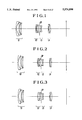•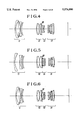•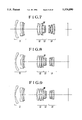•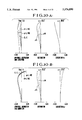•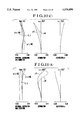•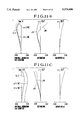•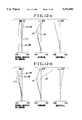•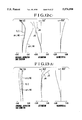•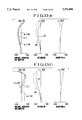•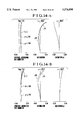••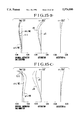•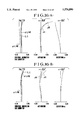•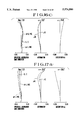•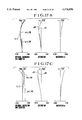•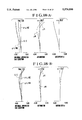•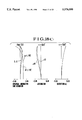•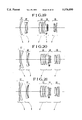•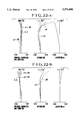•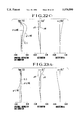•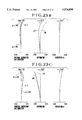•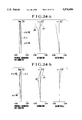•## Classifications

• GPHYSICS
• G02OPTICS
• G02BOPTICAL ELEMENTS, SYSTEMS, OR APPARATUS
• G02B15/00Optical objectives with means for varying the magnification
• G02B15/14Optical objectives with means for varying the magnification by axial movement of one or more lenses or groups of lenses relative to the image plane for continuously varying the equivalent focal length of the objective
• G02B15/16Optical objectives with means for varying the magnification by axial movement of one or more lenses or groups of lenses relative to the image plane for continuously varying the equivalent focal length of the objective with interdependent non-linearly related movements between one lens or lens group, and another lens or lens group
• G02B15/177Optical objectives with means for varying the magnification by axial movement of one or more lenses or groups of lenses relative to the image plane for continuously varying the equivalent focal length of the objective with interdependent non-linearly related movements between one lens or lens group, and another lens or lens group having a negative front lens or group of lenses
• GPHYSICS
• G02OPTICS
• G02BOPTICAL ELEMENTS, SYSTEMS, OR APPARATUS
• G02B15/00Optical objectives with means for varying the magnification
• G02B15/14Optical objectives with means for varying the magnification by axial movement of one or more lenses or groups of lenses relative to the image plane for continuously varying the equivalent focal length of the objective
• G02B15/144Optical objectives with means for varying the magnification by axial movement of one or more lenses or groups of lenses relative to the image plane for continuously varying the equivalent focal length of the objective having four groups only
• G02B15/1445Optical objectives with means for varying the magnification by axial movement of one or more lenses or groups of lenses relative to the image plane for continuously varying the equivalent focal length of the objective having four groups only the first group being negative
• G02B15/144511Optical objectives with means for varying the magnification by axial movement of one or more lenses or groups of lenses relative to the image plane for continuously varying the equivalent focal length of the objective having four groups only the first group being negative arranged -+-+
• GPHYSICS
• G02OPTICS
• G02BOPTICAL ELEMENTS, SYSTEMS, OR APPARATUS
• G02B15/00Optical objectives with means for varying the magnification
• G02B15/14Optical objectives with means for varying the magnification by axial movement of one or more lenses or groups of lenses relative to the image plane for continuously varying the equivalent focal length of the objective
• G02B15/145Optical objectives with means for varying the magnification by axial movement of one or more lenses or groups of lenses relative to the image plane for continuously varying the equivalent focal length of the objective having five groups only
• G02B15/1455Optical objectives with means for varying the magnification by axial movement of one or more lenses or groups of lenses relative to the image plane for continuously varying the equivalent focal length of the objective having five groups only the first group being negative
• G02B15/145521Optical objectives with means for varying the magnification by axial movement of one or more lenses or groups of lenses relative to the image plane for continuously varying the equivalent focal length of the objective having five groups only the first group being negative arranged --+-+

## Abstract

A zoom lens is disclosed comprising, from front to rear, a first lens unit of negative refractive power, a second lens unit of positive refractive power, a third lens unit of negative refractive power and a fourth lens unit of positive refractive power, zooming from a wide-angle end to a telephoto end being performed by moving at least the first, second and fourth lens units in such a way that an air separation between the first and second lens units decreases, an air separation between the second and third lens units increases and an air separation between the third and fourth lens units decreases, wherein the form and the construction and arrangement of the members of the lens units and, if an aspheric surface is in use, its shape, are appropriately determined.

## Description

This application is a continuation of application Ser. No. 08/022,156, filed Feb. 23, 1993, abandoned.
BACKGROUND OF THE INVENTION
1. Field of the Invention
This invention relates to zoom lenses suited to single-lens reflex cameras or video cameras and, more particularly, to zoom lenses of relatively wide angle, high range and large relative aperture of the type in which the front lens unit is negative in power, or the so-called "negative lead" type, having four or five lens units in total. Herein, the term "unit" is equivalent to "group".
2. Description of the Related Art
The negative lead type of zoom lens, because of the lens unit of negative refractive power coming first in arrangement, is easier to increase the maximum image angle and amenable to shorten the minimum possible object distance for close-up photography. With these merits on one hand, there are, on the other hand, drawbacks that the diameter of the stop is caused to increase and that the range of variation of the image magnification becomes difficult to increase.
To overcome these drawbacks, there have been made previous proposals for minimization of the bulk and size of the entirety of the lens system in combination with a great increase of the range, as in, for example, Japanese Patent Publications Nos. Sho 49-23912 and Sho 55-14403 and Japanese Laid-Open Patent Applications Nos. Sho 53-34539, Sho 57-163213, Sho 58-4113, Sho 63-241511, Hei 1-193709 and Hei 2-201310.
In each of these publications, the zoom lens is made to comprise four lens units of minus-plus-minus-plus power arrangement in this order from the object side as a whole, of which certain ones are moved in appropriate relation to vary the focal length.
Recently, for the single-lens reflex camera or video camera, the public is demanding a zoom lens which includes wide image angles and has a high zoom ratio as the standard equipment. For example, the market has already sold single-lens reflex cameras of 35 mm format having zoom lenses of as wide a maximum image angle as 35 mm or 28 mm in focal length with the telephoto end at 70 mm each as the standard equipment.
More recently, there is demanded a zoom lens having an extended range toward the telephoto end with the result of from 28 mm to 80 mm or to 85 mm in focal length and a large relative aperture.
As the field of view increases to so wide an angle and the range, too, extends to so long a focal length, however, it becomes necessary in general to admit an unduly large increase of the physical length of the zoom lens and moreover to make elaborate the relation in which the lens units move to effect zooming. This leads to a multi-sleeve construction of the lens mounting. Thus, a problem arises in that the lens barrel increases largely in bulk and size and its mounting mechanism comes to a very complicated structure.
SUMMARY OF THE INVENTION
According to the present invention, four lens units are used for constructing a complete zoom lens and appropriate conditions are defined for the refractive powers of the lens units and for the relation of movement of the lens units for zooming. Thus, the physical length of the lens is shortened and the lens mounting is prevented from increasing in size and from becoming complicated in structure. It is, therefore, an object of the invention to provide a zoom lens of relatively wide image angles having a high range, while still maintaining high optical performance throughout the entire zooming range.
A specific zoom lens of the invention has as large a relative aperture as 2.8 or thereabout in F-number, is nonetheless wide in angular field coverage, and has as high a range as approximately 2 to 3, and its optical performance is maintained stable at a high level throughout the entire zooming range.
Besides these features, in the present invention, the focusing provision is made not in the first lens unit but in any other, or the inner focus type is employed to make it easy to perform the focusing operation. Therefore, another object of the invention is to provide a wide angle zoom lens suited to be used in, for example, auto-focus cameras.
According to the invention, in a preferred embodiment thereof, a zoom lens comprises, from front to rear, a first lens unit of-negative refractive power, a second lens unit of positive refractive power, a third lens unit of negative refractive power and a fourth lens unit of positive refractive power, zooming from the wide-angle end to the telephoto end being performed by moving at least the first, second and fourth lens units in such a way that the air separation between the first and second lens units decreases, the air separation between the second and third lens units increases and the air separation between the third and fourth lens units decreases. Given this arrangement, the members in each lens unit and, if an aspheric surface is used, an equation for its shape, are properly figured.
With this zoom lens, further improvements are attained by dividing such a first lens unit into two parts of negative refractive powers which, when zooming, are made to move in respective different loci from each other.
In this regard, another embodiment is disclosed where of these divided parts, the rear negative one is used for focusing purposes.
These and other objects and features of the invention will become apparent from the following detailed description of preferred embodiments thereof with reference to the accompanying drawings.
BRIEF DESCRIPTION OF THE DRAWINGS
FIG. 1 is a longitudinal section view of a numerical example 1 of a zoom lens of the invention.
FIG. 2 is a longitudinal section view of a numerical example 2 of a zoom lens of the invention.
FIG. 3 is a longitudinal section view of a numerical example 3 of a zoom lens of the invention.
FIG. 4 is a longitudinal section view of a numerical example 4 of a zoom lens of the invention.
FIG. 5 is a longitudinal section view of a numerical example 5 of a zoom lens of the invention.
FIG. 6 is a longitudinal section view of a numerical example 6 of a zoom lens of the invention.
FIG. 7 is a longitudinal section view of a numerical example 7 of a zoom lens of the invention.
FIG. 8 is a longitudinal section view of a numerical example 8 of a zoom lens of the invention.
FIG. 9 is a longitudinal section view of a numerical example 9 of a zoom lens of the invention.
FIGS. 10(A), 10(B) and 10(C) are graphic representations of the various aberrations of the numerical example 1 of the invention in the wide-angle end, an intermediate position and the telephoto end, respectively.
FIGS. 11(A), 11(B) and 11(C) are graphic representations of the various aberrations of the numerical example 2 of the invention in the wide-angle end, an intermediate position and the telephoto end, respectively.
FIGS. 12(A), 12(B) and 12(C) are graphic representations of the various aberrations of the numerical example 3 of the invention in the wide-angle end, an intermediate position and the telephoto end, respectively.
FIGS. 13(A), 13(B) and 13(C) are graphic representations of the various aberrations of the numerical example 4 of the invention in the wide-angle end, an intermediate position and the telephoto end, respectively.
FIGS. 14(A), 14(B) and 14(C) are graphic representations of the various aberrations of the numerical example 5 of the invention in the wide-angle end, an intermediate position and the telephoto end, respectively.
FIGS. 15(A), 15(B) and 15(C) are graphic representations of the various aberrations of the numerical example 6 of the invention in the wide-angle end, an intermediate position and the telephoto end, respectively.
FIGS. 16(A), 16(B) and 16(C) are graphic representations of the various aberrations of the numerical example 7 of the invention in the wide-angle end, an intermediate position and the telephoto end, respectively.
FIGS. 17(A), 17(B) and 17(C) are graphic representations of the various aberrations of the numerical example 8 of the invention in the wide-angle end, an intermediate position and the telephoto end, respectively.
FIGS. 18(A), 18(B) and 18(C) are graphic representations of the various aberrations of the numerical example 9 of the invention in the wide-angle end, an intermediate position and the telephoto end, respectively.
FIG. 19 is a longitudinal section view of a numerical example 10 of a zoom lens of the invention.
FIG. 20 is a longitudinal section view of a numerical example 11 of a zoom lens of the invention.
FIG. 21 is a longitudinal section view of a numerical example 12 of a zoom lens of the invention.
FIGS. 22(A), 22(B) and 22(C) are graphic representations of the various aberrations of the numerical example 10 of the invention in the wide-angle end, an intermediate position and the telephoto end, respectively.
FIGS. 23(A), 23(B) and 23(C) are graphic representations of the various aberrations of the numerical example 11 of the invention in the wide-angle end, an intermediate position and the telephoto end, respectively.
FIGS. 24(A), 24(B) and 24(C) are graphic representations of the various aberrations of the numerical example 12 of the invention in the wide-angle end, an intermediate position and the telephoto end, respectively.
In these figures, S stands for the sagittal image surface, M for the meridional image surface, d for the spectral d-line, g for the spectral g-line, and SC for the sine condition.
DETAILED DESCRIPTION OF THE PREFERRED EMBODIMENTS
FIG. 1 to FIG. 9 in block diagrams show the numerical examples 1 to 9 of the invention, respectively. In these lens block diagrams, L1 denotes a first lens unit of negative refractive power, L2 a second lens unit of positive refractive power, L3 is a third lens unit of negative refractive power, and L4 is a fourth lens unit of positive refractive power. SP represents a stop.
In the invention, all the air separations between the lens units are made variable. In this respect, certain ones of the lens units are chosen to move for zooming purposes.
In particular, at least the first, second and fourth lens units are moved in such a way that, as zooming goes from the wide-angle end to the telephoto end, the air separation between the first and second lens units decreases, the air separation between the second and third lens units increases, and the air separation between the third and fourth lens units decreases. More specifically speaking, zooming from the wide-angle end to the telephoto end is performed by moving the first lens unit toward the image side, the second lens unit toward the object side and the fourth lens unit toward the object side.
In the second and fourth lens units, their forward movements may be made either independent of each other, or in unison with each other. In the latter case, simplification of the structure of the lens mounting is facilitated. Focusing is performed by moving the whole first lens unit. Besides this, as the first lens unit is divided into two lens sub-units, or a front lens sub-unit and a rear lens sub-unit, this rear lens sub-unit may otherwise be moved for focusing.
Next, the features of each of the numerical examples shown in FIG. 1 to FIG. 9 are described in order.
(i) In the numerical examples 1, 2 and 3 shown in FIGS. 1, 2 and 3, zooming from the wide-angle end to the telephoto end is performed by moving at least the first, second and fourth lens units in such a way that the air separation between the first and second lens units decreases, the air separation between the second and third lens units increases and the air separation between the third and fourth lens units decreases. The third lens unit is constructed from two separate lenses including a positive lens and negative lens, or from a cemented lens composed thereof, and the fourth lens unit is constructed from two separate lenses including a negative lens and a positive lens, or from a cemented lens composed thereof. Thus, the entire lens system gets a shorter total length than was heretofore possible, while still permitting a high optical performance to be obtained at the wide-angle end and moreover maintaining good stability of the aberrations throughout the entire zooming range.
The second lens unit is constructed as comprising, from front to rear, a negative lens of meniscus shape having a strong negative refracting surface facing the image side, a positive lens and a positive lens having a convex surface facing the object side. Thus, the second lens unit, though given a relatively strong positive refractive power, is well corrected for aberrations. Particularly on the telephoto side, the diameter of the light bundle emerging from the second lens unit is made smaller in order to insure that the diameter of the diaphragm aperture is shortened. Thus, the diameter of the outer barrel of the lens mounting is minimized.
The first lens unit is constructed from, front to rear, a negative lens of meniscus shape having a concave surface facing the image side, a negative lens and a positive lens of meniscus shape having a convex surface facing the object side. Commonly, a positive lens would be arranged at the frontmost position. In this case, distortion on the wide-angle side could be corrected well. However, the outer diameter of the first lens unit would be caused to increase largely.
According to the invention, therefore, the negative lens takes the frontmost position as described above in the construction of the first lens unit. So, the distortion is corrected by using the other lens units. Thus, the outer diameter of the first lens unit is minimized. In addition, for close-up photography, the possible minimum object distance is made sure to shorten.
The third lens unit is made stationary during zooming, thus contributing to a reduction of the complexity of structure of the mounting mechanism for the lens system. Further, the second and fourth lens units may move forward in unison with each other to vary the focal length from the wide-angle end to the telephoto end, so that a further reduction of the complexity of structure of the lens mounting mechanism can be achieved.
For the focal lengths F2 and F3 of the second and third lens units, respectively, the following relationship is set forth:
`F2<|F3|                                  (1-a)`
The use of the strong positive refractive power in the second lens unit assists in minimizing the diameter of the diaphragm aperture. Likewise as described above, this leads to a minimization of the diameter of the lens barrel. The third lens unit is, on the other hand, relatively weak in refractive power as compared with the second lens unit. This leads to a possibility of effectively correcting aberrations, though the third lens unit is constructed from only two lens elements.
For the focal length F4 of the fourth lens unit, the following relationship is set forth:
`0.5<F4/FT<2                                                (1-b)`
where FT is the focal length of the entire lens system at the telephoto end. This concerns good correction of aberrations with the limitation of the bulk and size of the entire lens system to a minimum.
When the refractive power of the fourth lens unit is too strong as exceeding the lower limit of the condition (1-b), the negative and positive lenses, or the two lens elements, become insufficient in number for the fourth lens unit to carry out good correction of aberrations. When the refractive power of the fourth lens unit is too weak as exceeding the upper limit, the total zooming movement of each of the lens units increases to increase the total length of the entire lens system objectionably.
(ii) In the numerical examples 4 and 5 shown in FIGS. 4 and 5, all the air separations between the lens units are varied to effect zooming. The second lens unit comprises a cemented lens composed of a negative first lens of meniscus shape having a convex surface facing the object side and a positive second lens whose surfaces both are convex, and a positive third lens having a strong positive refracting surface facing the object side. The front surface of the first or third lens is formed to aspheric shape. For the radius of curvature R2,2 of the cemented surface of the aforesaid cemented lens and the aspheric coefficients B and C of the aforesaid aspheric surface, the following conditions are set forth:
`0.6<R2,2/F2<1.0                                            (2-a)`
`B<0                                                        (2-b)`
`B+C×(DE/2).sup.2 <0                                  (2-c)`
where F2 is the focal length of the second lens unit, and DE is the outer diameter of the third lens. When these conditions are satisfied, the entire lens system has its total length shortened, and the various aberrations are well corrected for a high optical performance throughout the entire zooming range.
In the present embodiment, from the first lens unit of negative refractive power, a diverging light beam emerges and enters the second lens unit. For this reason, as the F-number becomes faster, the light beam is incident on the second lens unit at a higher position from the optical axis. As a result, the marginal zone of the second lens unit produces very large aberrations, thus lowering the optical performance.
As the countermeasure to this, mention may be made of the methods of increasing the number of lens elements in the second lens unit, and of using a weakened refractive power in the second lens unit. These methods are, however, not favorable, because the total length of the complete lens becomes long and the outer diameter of the first lens unit is increased.
Therefore, in the present embodiment, the second lens unit which would otherwise produce various aberrations of large magnitude, particularly spherical and comatic ones, is well corrected by the three lenses which satisfy the above-cited conditions (2-a), (2-b) and (2-c) and the aspheric surface. Thus, a high optical performance is obtained.
The inequalities of condition (2-a) have an aim chiefly to correct coma. When the lower limit of condition (2-a) is exceeded, over-correction of coma results. Conversely when the upper limit is exceeded, it becomes difficult to correct the residual coma by using the other surfaces, and to do good correction of it together with spherical aberration and astigmatism. Further, it becomes difficult to correct all aberrations of the entire lens system in good balance.
The inequalities of conditions (2-b) and (2-c) have an aim to determine an appropriate aspheric shape to well correct, in particular, spherical aberration and coma. Of these, the factor in the condition (2-b) represents the aberration component of third order. This is taken negative. That is, the positive refractive power gets weaker in the marginal zone. By this function, the spherical aberration and coma are shifted in the over-correcting direction. In combination with the condition (2-a), therefore, good correction of spherical aberration and coma is attained.
The inequality of condition (2-c) takes into account the aberration components of up to 5th orders when the appropriate aspheric shape is determined. So, the aspheric amount at the position of the outer diameter DE of the lens is determined so as to weaken the refractive power in the marginal zone. Thus, the various aberrations produced are reduced, although the relatively strong positive refractive power is laid on the second lens unit. This gives rise to an additional advantage that the first lens unit can take a strong negative refractive power so that a shortening of the total length of the complete lens is achieved.
Besides these, the numerical example 5 has its first lens unit to include at least two negative lenses and positive lenses. By this, astigmatism and distortion are effectively corrected.
The first lens unit comprises, from the object side, a front lens sub-unit and a rear lens sub-unit, totaling two lens sub-units, of which the rear lens sub-unit is made axially movable for focusing. During the focusing operation, therefore, the total length of the complete lens remains constant. Since the lens does not protrude forward, and the rear lens sub-unit that is used for focusing is relatively small in size and light in weight, when in application to, for example, the automatic focusing camera, the drive control becomes easy to do.
(iii) In the numerical examples 6 and 7 shown in FIGS. 6 and 7, all the air separations between the lens units are varied when zooming. The second lens unit includes a cemented lens composed of a negative first lens of meniscus shape having a convex surface facing the object side and a positive second lens whose surfaces both are convex, and a positive third lens having a strong positive refracting surface facing the object side. The rear surface of the second or third lens is formed to aspheric shape. For the radius of curvature R2,2 of the cemented surface of the aforesaid cemented lens and the aspheric coefficients B and C of the aforesaid aspheric surface, the following conditions are set forth:
`0.6<R2,2/F2<1.0                                            (3-a)`
`B>0                                                        (3-b)`
`B+C×(DE/2).sup.2 >0                                  (3-c)`
where F2 is the focal length of the second lens unit and DE is the outer diameter of the third lens. When these conditions are satisfied, similar results to those of the numerical examples 4 and 5 described before are effected. That is, while a shortening of the total length of the entire lens system is achieved, all aberrations with zooming are well corrected, so that a high optical performance is obtained throughout the entire zooming range.
In the present embodiment, from the first lens unit of negative refractive power to the second lens unit, the emerging light beam is diverging. For this reason, the faster the F-number, the higher the height of incidence on the second lens unit from the optical axis. This results in production of very large aberrations in the marginal zone of the second lens unit, which lowers the optical performance.
To prevent this, the number of lens elements in the second lens unit may be increased, or the refractive power of the second lens unit may be weakened. These methods are, however, associated with drawbacks of elongating the total length of the entire lens system and of increasing the outer diameter of the first lens unit.
In the present embodiment, therefore, the second lens unit whose various aberrations, particularly spherical aberration and coma, would otherwise increase largely is well corrected by the three lenses which satisfy the above-cited conditions (3-a), (3-b) and (3-c) and the aspheric surface. Thus, a high optical performance is obtained.
The inequalities of condition (3-a) have an aim chiefly to correct coma. When the lower limit of the condition (3-a) is exceeded, over-correction of coma results. Conversely when the upper limit is exceeded, it becomes difficult to correct the residual coma by using any other surfaces and to do good correction of it together with spherical aberration and astigmatism. Further, it also becomes difficult to correct all aberrations of the entire lens system in good balance.
The inequalities of conditions (3-b) and (3-c) give the aspheric surface an appropriate shape to well correct, in particular, spherical aberration and coma. Of these, the condition (3-b) gives negative sign to the aberration component of third order, in other words, produces a function of weakening the positive refractive power in the marginal zone. Thus, the spherical aberration and coma are over-corrected. In combination with the condition (3-a), therefore, good correction of spherical aberration and coma results.
The inequality of condition (3-c) brings the aberration components of up to fifth orders in determining an appropriate shape of the aspheric surface to control the aspheric amount particular at the position of the outer diameter DE of the lens so that the refractive power is weakened in the marginal zone. Despite the second lens unit having its positive refractive power made relatively strong, therefore, the various aberrations are reduced. This also allows the first lens unit to be given a strong negative refractive power with an advantage of achieving a shortening of the total length of the complete lens.
Besides these, the numerical example 7 has another feature that, similarly to the numerical examples 4 and 5 described before, the first lens unit includes at least two negative lenses and a positive lens. By this, astigmatism and distortion are corrected well.
Again, the first lens unit comprises, from the object side, a front lens sub-unit and a rear lens sub-unit, totaling two lens sub-units, of which the rear lens sub-unit is made axially movable for focusing. Thus, the total length of the complete lens remains constant during focusing. So, the lens does not protrude outward. Again, because the rear lens sub-unit to move is relatively small in size and light in weight, the driving control in, for example, automatic focus mode is made quick and easy.
(iv) In the numerical examples 8 and 9 shown in FIGS. 8 and 9, zooming from the wide-angle end to the telephoto end is performed by decreasing the air separation between the first and second lens units, increasing the air separation between the second and third lens units, and decreasing the air separation between the third and fourth lens units. The fourth lens unit includes at least one negative lens and at least one positive lens and has at least one aspheric surface. Letting the refractive power of the aspheric surface be denoted by φ4a, the radius of curvature of a sphere defined by the intersection point of the aspheric surface with the optical axis and the circle of maximum diameter on the aspheric surface by Rmax, and the radius of curvature of a sphere defined by the intersection point of the aspheric surface with the optical axis and a circle on the aspheric surface at a height H from the optical axis by RH, the following condition is satisfied:
`(|Rmax|-|RH|)·φ4a<0(4-a)`
Despite the increases of the range and relative aperture, this assures maintenance of high optical performance throughout the entire zooming range.
In the present embodiment, the first lens unit is made negative in refractive power to afford an advantageous lens configuration to the increase of the maximum image angle. Furthermore, in addition to the first and second lens units, the third and fourth lens units are used to share the variation of the focal length. Thus, it is made easy to achieve a great increase of the zooming range.
In another general rule, for a reduction of the bulk and size of the entire lens system under the condition of the high range and the large relative aperture, there is need to strengthen the refractive power of each of the lens units. With this, large aberrations are produced. So, the number of lens elements has to be increased for correcting purposes.
In the present embodiment, therefore, the form and the arrangement of the members of the fourth lens unit are properly determined. Particularly with the use of the aspheric surface in combination, a small number of lens members is sufficient for the spherical aberration and astigmatism and others to be corrected well.
The inequality of condition (4-a) gives to the aspheric surface in the fourth lens unit a shape that the positive refractive power gets progressively weaker as the height from the optical axis increases to thereby effectively correct mainly aspherical aberration and astigmatism. When the condition (4-a) is violated, the aspherical aberration and astigmatism increase largely. To correct these, the number of members of the fourth lens unit must be increased. As a result, the total length of the complete lens becomes long objectionably.
Besides these, the numerical examples of the present embodiment have their first lens units included with at least two negative lenses and a positive lens. This simultaneously fulfills the requirements of shortening the shortest focal length to achieve the increase of the angle of field of view and of minimizing the outer diameter of the first lens unit. In addition, good correction of astigmatism and distortion is attained.
The second lens unit includes a cemented lens composed of a negative first lens of meniscus shape having a convex surface facing the object side and a positive second lens whose surfaces both are convex and a positive third lens having a strong positive refracting surface facing the object side, wherein the following condition is satisfied:
`0.6<R2,2/F2<1.0                                            (4-b)`
where R2,2 is the radius of curvature of the cemented surface of the cemented lens, and F2 is the focal length of the second lens unit.
Since, in the present embodiment, the first lens unit has a negative refractive power, the axial light beam arrives on the second lens unit at a higher position from the optical axis than with the first lens unit. For this reason, aberrations are liable to be generated. So, the members of the second lens unit are constructed and arranged in the ways described above. Thus, a good correction of various aberrations is attained.
The inequalities of condition (4-b) give a proper range for the radius of curvature of the cemented surface of the cemented lens in order to effectively correct mainly coma. When the radius of curvature R2,2 is made smaller as exceeding the lower limit of condition (4-b), over-correction of coma results. Conversely when the radius of curvature R2,2 is made larger as exceeding the upper limit, it becomes difficult to correct the residual coma by using any other surfaces.
The numerical data for the specific examples 1 to 9 of the invention are shown below. In the tables of the examples 1 to 9, Ri is the radius of curvature of the i-th lens surface when counted from the object side, Di is the i-th lens thickness or air separation when counted from the object side, and Ni and υi are respectively the refractive index and Abbe number of the glass of the i-th lens element when counted from the object side.
The shape of an aspheric surface is expressed with the coordinates with an X axis in the axial direction and an H axis in the perpendicular direction to the optical axis, the direction in which light advances being taken positive, by the following equation: ##EQU1## where R is the radius of the osculating sphere, and A, B, C, D and E are the aspheric coefficients.
Numerical Example 1:
(FIGS. 1, 10(A), 10(B)and 10(C))
`__________________________________________________________________________F = 28.8-77.3 FNO = 1:3.5-4.5 2ω = 73.8°-31.3°__________________________________________________________________________R 1 = 57.039 D 1 = 1.80                 N 1 = 1.77250                         ν 1 = 49.6R 2 = 23.561 D 2 = 6.90R 3 = -13365.018        D 3 = 1.60                 N 2 = 1.77250                         ν 2 = 49.6R 4 = 40.913 D 4 = 0.10R 5 = 30.586 D 5 = 3.90                 N 3 = 1.80518                         ν 3 = 25.4R 6 = 65.175 D 6 = VariableR 7 = 61.327 D 7 = 1.50                 N 4 = 1.84666                         ν 4 = 23.9R 8 = 25.956 D 8 = 0.07R 9 = 27.135 D 9 = 4.00                 N 5 = 1.69680                         ν 5 = 55.5R10 = -157.956        D10 = 0.10R11 = 35.052 D11 = 3.15                 N 6 = 1.69680                         ν 6 = 55.5R12 = -166.755        D12 = VariableR13 = (Stop) D13 = 1.75R14 = -51.650        D14 = 2.10                 N 7 = 1.80518                         ν 7 = 25.4R15 = -25.090        D15 = 1.30                 N 8 = 1.60311                         ν 8 = 60.7R16 = 46.084 D16 = VariableR17 = 122.517        D17 = 1.30                 N 9 = 1.80518                         ν 9 = 25.4R18 = 31.966 D18 = 0.42R19 = 35.150 D19 = 5.00                 N10 = 1.58313                         ν10 = 59.4R20 = -37.015 (Aspheric)R21 = 0.0__________________________________________________________________________`
`______________________________________Variable    Focal LengthSeparation  28.80        52.36  77.30______________________________________D 6         40.37        11.65   1.03D12          2.14        10.74  19.34D16         18.91        10.31   1.71______________________________________`
R20: Aspheric Surface
The Values of the Aspheric Coefficients
`______________________________________A = 0              B = 4.88934 × 10.sup.-6C = -6.38115 × 10.sup.-9              D = 1.53483 × 10.sup.-1E = -5.17930 × 10.sup.-13______________________________________`
Numerical Example 2:
(FIGS. 2, 11(A), 11(B) and 11(C))
`__________________________________________________________________________F = 28.8-77.01 FNO = 1:3.5-4.5 2ω = 73.8°-31.4°__________________________________________________________________________R 1 = 69.290 D 1 = 1.80                 N 1 = 1.77250                         ν 1 = 49.6R 2 = 26.715 D 2 = 6.90R 3 = 3674.837        D 3 = 1.60                 N 2 = 1.77250                         ν 2 = 49.6R 4 = 37.386 D 4 = 0.10R 5 = 31.958 D 5 = 3.90                 N 3 = 1.80518                         ν 3 = 25.4R 6 = 69.851 D 6 = VariableR 7 = 62.034 D 7 = 1.50                 N 4 = 1.84666                         ν 4 = 23.9R 8 = 25.952 D 8 = 0.07R 9 = 26.641 D 9 = 4.00                 N 5 = 1.69680                         ν 5 = 55.5R10 = -110.081        D10 = 0.10R11 = 31.365 D11 = 3.15                 N 6 = 1.69680                         ν 6 = 55.5R12 = -395.214        D12 = VariableR13 = (Stop) D13 = 1.75R14 = -55.195        D14 = 2.10                 N 7 = 1.80518                         ν 7 = 25.4R15 = -25.214        D15 = 1.30                 N 8 = 1.60311                         ν 8 = 60.7R16 = 38.462 D16 = VariableR17 = 78.946 D17 = 1.30                 N 9 = 1.80518                         ν 9 = 25.4R18 = 26.654 D18 = 0.42R19 = 29.350 D19 = 5.00                 N10 = 1.58313                         ν10 = 59.4R20 = -44.797 (Ashperic)R21 = 0.0__________________________________________________________________________`
`______________________________________Variable    Focal LengthSeparation  28.80        52.30  77.01______________________________________D 6         39.81        11.56   1.01D12          2.34        10.34  18.34D16         17.95         9.95   1.95______________________________________`
R20: Aspheric Surface
The Values of the Aspheric Coefficients:
`______________________________________A = 0              B = 4.54988 × 10.sup.-6C = 3.91367 × 10.sup.-9              D = 1.11697 × 10.sup.-10E = -6.30557 × 10.sup.-13______________________________________`
Numeral Example 3:
(FIGS. 3, 12(A), 12(B)and 12(C))
`__________________________________________________________________________F = 28.8-77.38 FNO = 1:3.5-4.5 2ω = 73.8°-31.2°__________________________________________________________________________R 1 = 67.090 D 1 = 1.80                 N 1 = 1.77250                         ν 1 = 49.6R 2 = 27.602 D 2 = 6.80R 3 = -912.555        D 3 = 1.60                 N 2 = 1.77250                         ν 2 = 49.6R 4 = 36.134 D 4 = 0.10R 5 = 31.918 D 5 = 3.90                 N 3 = 1.80518                         ν 3 = 25.4R 6 = 73.004 D 6 = VariableR 7 = 48.711 D 7 = 1.50                 N 4 = 1.84666                         ν 4 = 23.9R 8 = 22.792 D 8 = 4.00                 N 5 = 1.69680                         ν 5 = 55.5R 9 = -89.964        D 9 = 0.10R10 = 31.279 D10 = 3.10                 N 6 = 1.69680                         ν 6 = 55.5R11 = 248.913        D11 = VariableR12 = (Stop) D12 = 1.75R13 = -51.342        D13 = 2.10                 N 7 = 1.80518                         ν 7 = 25.4R14 = -23.117        D14 = 1.30                 N 8 = 1.60311                         ν 8 = 60.7R15 = 38.796 D15 = VariableR16 = 76.641 D16 = 1.30                 N 9 = 1.84666                         ν 9 = 23.9R17 = 29.240 D17 = 0.45R18 = 32.398 D18 = 5.00                 N10 = 1.5813                         ν10 = 59.4R19 = -45.125 (Aspheric)__________________________________________________________________________`
`______________________________________Variable    Focal LengthSeparation  28.80        52.46  77.38______________________________________D 6         40.21        12.05   1.58D11          1.96         9.96  17.96D15         17.67         9.67   1.67______________________________________`
R19: Aspheric Surface
The Values of the Aspheric Coefficients:
`______________________________________A = 0              B = 4.7416 × 10.sup.-6C = 3.16871 × 10.sup.-8              D = -1.19894 × 10.sup.-10E = 2.29582 × 10.sup.-13______________________________________`
Numerical Example 4:
(FIGS. 4, 13(A), 13(B)and 13(C))
`__________________________________________________________________________F = 28.8-77.49 FNO = 1:2.8 2ω = 73.8°-31.2°__________________________________________________________________________R 1 = 90.226 D 1 = 2.00                 N 1 = 1.77250                         ν 1 = 49.6R 2 = 38.296 D 2 = 9.00R 3 = -461.888        D 3 = 4.50                 N 2 = 1.69895                         ν 2 = 30.1R 4 = -96.235        D 4 = 0.12R 5 = -179.098        D 5 = 1.80                 N 3 = 1.77250                         ν 3 = 49.6R 6 = 59.711 D 6 = 1.70R 7 = 39.696 D 7 = 2.90                 N 4 = 1.84666                         ν 4 = 23.9R 8 = 48.254 D 8 = VariableR 9 = 61.886 D 9 = 1.50                 N 5 = 1.84666                         ν 5 = 23.8R10 = 36.536 D10 = 7.00                 N 6 = 1.71300                         ν 6 = 53.8R11 = -92.015        D11 = 0.10R12 = 43.139 (Aspheric)        D12 = 5.50                 N 7 = 1.77250                         ν 7 = 49.6R13 = -469.393        D13 = 1.50                 N 8 = 1.84666                         ν 8 = 23.8R14 = 129.333        D14 = VariableR15 = (Stop) D15 = 1.00R16 = 805.385        D16 = 4.10                 N 9 = 1.84666                         ν 9 = 23.8R17 = -23.912        D17 = 1.10                 N10 = 1.77250                         ν10 = 49.6R18 = 56.787 D18 = 2.80R19 = -32.304        D19 = 1.10                 N11 = 1.83481                         ν11 = 42.7R20 = -279.112        D20 = VariableR21 = -61.974        D21 = 1.20                 N12 = 1.84666                         ν12 = 23.8R22 = 82.543 D22 = 3.80                 N13 = 1.71300                         ν13 = 53.8R23 = -34.909        D23 = 0.10R24 = 49.546 D24 = 1.80                 N14 = 1.76182                         ν14 = 26.5R25 = 39.572 D25 = 3.50R26 = -706.308        D26 = 2.80                 N15 = 1.69680                         ν15 = 55.5R27 = -64.519        D27 = 0.10R28 = 77.104 D28 = 4.80                 N16 = 1.71300                         ν16 = 53.8R29 = -104.361        D29 = 1.40                 N17 = 1.84666                         ν17 = 23.8R30 = -193.661__________________________________________________________________________`
`______________________________________Variable    Focal LengthSeparation  28.80        52.29  77.49______________________________________D 8         48.37        14.88   2.28D14          1.82        11.82  21.82D20         10.45        6.0     1.56______________________________________`
R12: Aspheric Surface
The Values of the Aspheric Coefficients:
`______________________________________A = 0              B = -1.67796 × 10.sup.-7C = -1.68935 × 10.sup.-9              D = -1.43301 × 10.sup.-12______________________________________`
Numerical Example 5:
(FIGS. 5, 14(A), 14(B) and 14(C))
`__________________________________________________________________________F = 28.8-77.63 FNO = 1:2.8 2ω = 73.8°-31.1°__________________________________________________________________________R 1 = 107.701 (Aspheric)        D 1 = 2.20                 N 1 = 1.83481                         ν 1 = 42.7R 2 = 43.329 D 2 = 16.00R 3 = -663.644        D 3 = 2.00                 N 2 = 1.83481                         ν 2 = 42.7R 4 = 35.965 D 4 = 5.00                 N 3 = 1.84666                         ν 3 = 23.8R 5 = 96.556 D 5 = 1.50R 6 = 56.255 D 6 = 2.50                 N 4 = 1.84666                         ν 4 = 23.8R 7 = 62.609 D 7 = VariableR 8 = 78.988 (Aspheric)        D 8 = 1.50                 N 5 = 1.84666                         ν 5 = 23.8R 9 = 39.104 D 9 = 7.50                 N 6 = 1.71300                         ν 6 = 53.8R10 = -85.722        D10 = 0.10R11 = 41.699 D11 = 7.00                 N 7 = 1.77250                         ν 7 = 49.6R12 = -136.277        D12 = 1.50                 N 8 = 1.84666                         ν 8 = 23.8R13 = 183.298        D13 = VariableR14 = (Stop) D14 = 1.00R15 = 413.138        D15 = 4.10                 N 9 = 1.84666                         ν 9 = 23.8R16 = -27.684        D16 = 1.10                 N10 = 1.77250                         ν10 = 49.6R17 = 53.170 D17 = 2.80R18 = -32.983        D18 = 1.10                 N11 = 1.83481                         ν11 = 42.7R19 = -4847.456        D19 = VariableR20 = -80.792        D20 = 1.20                 N12 = 1.84666                         ν12 = 23.8R21 = -461.613        D21 = 3.50                 N13 = 1.71300                         ν13 = 53.8R22 = -36.396        D22 = 0.10R23 = 45.586 D23 = 1.80                 N14 = 1.76182                         ν14 = 26.5R24 = 37.053 D24 = 3.50R25 = -159.162        D25 = 2.50                 N15 = 1.69680                         ν15 = 55.5R26 = -56.467        D26 = 0.10R27 = 95.167 D27 = 5.00                 N16 = 1.71300                         ν16 = 53.8R28 = -47.606        D28 = 1.40                 N17 = 1.84666                         ν17 = 23.8R29 = -121.554__________________________________________________________________________`
`______________________________________Variable    Focal LengthSeparation  28.8         51.91  77.63______________________________________D 7         44.57        13.71   1.67D13          1.29        11.29  21.29D19         10.32         5.88   1.44______________________________________`
R1: Aspheric Surface
The values of the Aspheric Coefficients:
`______________________________________A = 0              B = 7.15543 × 10.sup.-7C = -2.45904 × 10.sup.-10              D = -1.41751 × 10.sup.-13______________________________________`
R8: Aspheric Surface
The Values of the Aspheric Coefficients:
`______________________________________The Values of the Aspheric Coefficients:______________________________________A = 0           B = 3.12771 × 10.sup.-7C = 9.59892 × 10.sup.-11______________________________________`
Numerical Example 6:
(FIGS. 6, 15(A), 15(B) and 15(C))
`______________________________________F = 28.8-77.43  FNO = 1:2.8  2ω = 73.8°-31.2°______________________________________R1 = 72.775      D 1 = 2.00 N 1 = 1.77250                              ν 1 = 49.6R 2 = 37.706      D 2 = 9.00R 3 = -474.784      D 3 = 4.50 N 2 = 1.69895                              ν 2 = 30.1R 4 = -94.031      D 4 = 0.12R 5 = -151.662      D 5 = 1.80 N 3 = 1.77250                              ν 3 = 49.6R 6 = 60.031      D 6 = 1.70R 7 = 40.187      D 7 = 2.90 N 4 = 1.84666                              ν 4 = 23.9R 8 = 46.071      D 8 = VariableR 9 = 77.426      D 9 = 1.50 N 5 = 1.84666                              ν 5 = 23.8R10 = 35.026      D10 = 7.00 N 6 = 1.71300                              ν 6 = 53.8R11 = -89.434      D11 = 0.10(Aspheric)R12 = 39.339      D12 = 5.50 N 7 = 1.77250                              ν 7 = 49.6R13 = 244.065      D13 = 1.50 N`18 = 1.84666                              ν 8 = 23.8R14 = 139.168      D14 = VariableR15 = (Stop)      D15 = 1.00R16 = 289.330      D16 = 4.10 N 9 = 1.84666                              ν 9 = 23.8R17 = -24.625      D17 = 1.10 N10 = 1.77250                              ν10 = 49.6R18 = 54.408      D18 = 2.80R19 = -31.708      D19 = 1.10 N11 = 1.83481                              ν11 = 42.7R20 = -632.173      D20 = VariableR21 = -58.935      D21 = 1.20 N12 = 1.84666                              ν12 = 23.8R22 = 83.573      D22 = 3.80 N13 = 1.71300                              ν13 = 53.8R23 = -33.512      D23 = 0.10R24 = 48.387      D24 = 1.80 N14 = 1.76182                              ν14 = 26.5R25 = 39.391      D25 = 3.50R26 = 263.616      D26 = 2.80 N15 = 1.69680                              ν15 = 55.5R27 = -73.712      D27 = 0.10R28 = 79.107      D28 = 4.80 N16 = 1.71300                              ν16 = 53.8R29 = -103.977      D29 = 1.40 N17 = 1.84666                              ν17 = 23.8R30 = -302.891______________________________________`
`______________________________________Variable   Focal LengthSeparation 28.8         52.13  77.43______________________________________D 8        46.71        14.46  2.24D14        1.8          11.8   21.8D20        10.5         6.06   1.62______________________________________`
R11: Aspheric Surface
`______________________________________A = 0              B = -3.75089 × 10.sup.-7C = 2.89669 × 10.sup.-10              D = 6.62424 × 10.sup.-13______________________________________`
Numerical Example 7:
(FIGS. 7, 16(A), 16(B)and 16(C))
`______________________________________F = 28.8-77.4  FNO = 1:2.8  2ω = 73.8°-31.2°______________________________________R 1 = 112.318      D 1 = 2.20 N 1 = 1.83481                              ν 1 = 42.7(Aspheric)R 2 = 43.560      D 2 = 16.00R 3 = -1512.328      D 3 = 2.00 N 2 = 1.83481                              ν 2 = 42.7R 4 = 35.617      D 4 = 5.00 N 3 = 1.84666                              ν 3 = 23.8R 5 = 88.280      D 5 = 1.50R 6 = 53.563      D 6 = 2.50 N 4 = 1.84666                              ν 4 = 23.8R 7 = 60.304      D 7 = VariableR 8 = 79.550      D 8 = 1.50 N 5 = 1.84666                              ν 5 = 23.8R 9 = 39.269      D 9 = 7.50 N 6 = 1.71300                              ν 6 = 53.8R10 = -88.346      D10 = 0.10R11 = 41.335      D11 = 7.00 N 7 = 1.77250                              ν 7 = 49.6R12 = -147.232      D12 = 1.50 N 8 = 1.84666                              ν 8 = 23.8R13 = 188.635      D13 = Variable(Aspheric)R14 = (Stop)      D14 = 1.00R15 = 662.981      D15 = 4.10 N 9 = 1.84666                              ν 9 = 23.8R16 = -26.757      D16 = 1.10 N10 = 1.77250                              ν10 = 49.6R17 = 55.441      D17 = 2.80R18 = -33.069      D18 = 1.10 N11 = 1.83481                              ν11 = 42.7R19 = -7905.451      D19 = VariableR20 = -83.147      D20 = 1.20 N12 = 1.84666                              ν12 = 23.8R21 = 883.745      D21 = 3.50 N13 = 1.71300                              ν13 = 53.8R22 = -36.241      D22 = 0.10R23 = 45.804      D23 = 1.80 N14 = 1.76182                              ν14 = 26.5R24 = 37.229      D24 = 3.50R25 = -182.280      D25 = 2.50 N15 = 1.69680                              ν15 = 55.5R26 = -57.812      D26 = 0.10R27 = 87.431      D27 = 5.00 N16 = 1.71300                              ν16 = 53.8R28 = -56.322      D28 = 1.40 N17 = 1.84666                              ν17 = 23.8R29 = -149.333______________________________________`
`______________________________________Variable   Focal LengthSeparation 28.8         51.81  77.4______________________________________D 7        44.55        13.81  1.79D13        1.78         11.78  21.78D19        10.59        6.14   1.7______________________________________`
R1: Aspheric Surface
The Values of the Aspheric Coefficients:
`______________________________________A = 0              B = 5.78991 × 10.sup.-7C = -4.62210 × 10.sup.-11              D = 1.60066 × 10.sup.-14______________________________________`
R13: Aspheric Surface
The Values of the Aspheric Coefficients:
`______________________________________A = 0              B = 3.56175 × 10.sup.-7C = -1.61754 × 10.sup.-11              D = 1.481666 × 10.sup.-13______________________________________`
Numerical Example 8:
(FIGS. 8, 17(A), 17(B) and 17(C))
`______________________________________F = 28.8-77.46  FNO = 1:2.8  2ω = 73.8°-31.2°______________________________________R 1 = 75.223      D 1 = 2.20 N 1 = 1.83481                              ν 1 = 42.7(Aspheric)R 2 = 37.278      D 2 = 16.00R 3 = -1600.958      D 3 = 2.00 N 2 = 1.83481                              ν 2 = 42.7R 4 = 32.493      D 4 = 5.50 N 3 = 1.84666                              ν 3 = 23.8R 5 = 71.677      D 5 = 1.50R 6 = 50.112      D 6 = 3.00 N 4 = 1.84666                              ν 4 = 23.8R 7 = 61.032      D 7 = VariableR 8 = 74.083      D 8 = 1.50 N 5 = 1.84666                              ν 5 = 23.8R 9 = 32.486      D 9 = 7.50 N 6 = 1.71300                              ν 6 = 53.8R10 = -289.769      D10 = 0.10R11 = 76.844      D11 = 5.50 N 7 = 1.77250                              ν 7 = 49.6R12 = -151.673      D12 = 1.40 N 8 = 1.84666                              ν 8 = 23.8R13 = 340.344      D13 = 0.10R14 = 54.698      D14 = 4.50 N 9 = 1.77250                              ν 9 = 49.6R15 = 1076.398      D15 = VariableR16 = (Stop)      D16 = 1.00R17 = 179.919      D17 = 4.10 N10 = 1.84666                              ν10 = 23.8R18 = -29.320      D18 = 1.10 N11 = 1.77250                              ν11 = 49.6R19 = 55.441      D19 = 2.90R20 = -32.601      D20 = 1.10 N12 = 1.83481                              ν12 = 42.7R21 = 199.184      D21 = VariabaleR22 = -146.326      D22 = 1.40 N13 = 1.84666                              ν13 = 23.8R23 = -238.520      D23 = 3.80 N14 = 1.71300                              ν14 = 53.8R24 = -31.546      D24 = 0.10R25 = -92.279      D25 = 2.80 N15 = 1.69680                              ν15 = 55.5R26 = -56.757      D26 = 0.10R27 = 125.405      D27 = 5.00 N16 = 1.71300                              ν16 = 53.8(Aspheric)R28 = -38.566      D28 = 1.40 N17 = 1.84666                              ν17 = 23.8R29 = -373.726______________________________________`
`______________________________________Variable   Focal LengthSeparation 28.8         51.51  77.46______________________________________D 7        42.41        13.39  1.74D15        1.78         11.78  21.78D21        10.68        6.23   1.79______________________________________`
R1: Aspheric Surface
The Values of the Aspheric Coefficients:
`______________________________________A = 0              B = 3.78122 × 10.sup.-7C = 2.31162 × 10.sup.-10              D = -7.07093 × 10.sup.-14______________________________________`
R27: Aspheric Surface
The Values of the Aspheric Coefficients
`______________________________________A = 0              B = -1.9333 × 10.sup.-6C = -4.86862 × 10.sup.-9              D = 3.0832 × 10.sup.-12______________________________________`
Numerical Example 9:
(FIGS. 9, 18(A), 18(B)and 18(C))
`______________________________________F = 28.8-77.35  FNO = 1:2.8  2ω = 73.8°-31.2°______________________________________R 1 = 82.212      D 1 = 2.20 N 1 = 1.83481                             ν 1 = 42.7(Aspheric)R 2 = 40.145      D 2 = 16.00R 3 = -734.079      D 3 = 2.00 N 2 = 1.83481                             ν 2 = 42.7R 4 = 32.424      D 4 = 5.50 N 3 = 1.84666                             ν 3 = 23.8R 5 = 65.788      D 5 = 1.50R 6 = 49.287      D 6 = 3.00 N 4 = 1.84666                             ν 4 = 23.8R 7 = 61.693      D 7 = Vari-      ableR 8 = 77.960      D 8 = 1.50 N 5 = 1.84666                             ν 5 = 23.8R 9 = 31.802      D 9 = 7.50 N 6 = 1.71300                             ν 6 = 53.8R10 = -198.781      D10 = 0.10R11 = 77.219      D11 = 5.50 N 7 = 1.77250                             ν 7 = 49.6R12 = -168.989      D12 = 1.40 N 8 = 1.84666                             ν 8 = 23.8R13 = 357.536      D13 = 0.10R14 = 50.032      D14 = 4.50 N 9 = 1.77250                             ν 9 = 49.6R15 = 1036.923      D15 = Vari-      ableR16 = (Stop)      D16 = 1.00R17 = 159.183      D17 = 4.10 N10 = 1.84666                             ν10 = 23.8R18 = -28.287      D18 = 1.10 N11 = 1.77250                             ν11 = 49.6R19 = 53.088      D19 = 2.90R20 = -34.394      D20 = 1.10 N12 = 1.83481                             ν12 = 42.7R21 = 122.601      D21 = Vari-      ableR22 = -152.863      D22 = 1.40 N13 = 1.84666                             ν13 = 23.8R23 = -275.361      D23 = 3.80 N14 = 1.71300                             ν14 = 53.8R24 = -31.874      D24 = 0.10R25 = -92.992      D25 = 2.80 N15 = 1.69680                             ν15 = 55.5R26 = -57.447      D26 = 0.10R27 = 126.107      D27 = 5.00 N16 = 1.71300                             ν16 = 53.8R28 = -38.422      D28 = 1.40 N17 = 1.84666                             ν17 = 23.8R29 = -550.494(Aspheric)______________________________________`
`______________________________________Variable    Focal LengthSeparation  28.8         51.47  77.35______________________________________D 7         42.81        13.44  1.65D15         1.95         10.8   19.65D21         11.11        6.26   1.41______________________________________`
R1: Aspheric Surface
The Values of the Aspheric Coefficients:
`______________________________________A = 0             B = 1.90348 × 10.sup.-7C = 2.99605 × 10.sup.-10             D = -1.28883 × 10.sup.-13______________________________________`
R29: Aspheric Surface
The Values of the Aspheric Coefficients:
`______________________________________A = 0             B = 1.86774 × 10.sup.-6C = 6.79916 × 10.sup.-9             D = -7.60901 × 10.sup.-12______________________________________`
According to the above-described numerical examples, the design rules for the refractive powers of the four lens units and the relation in which the lens units move with zooming are set forth as described before. By these rules, the total length of the complete lens is shortened and the mounting mechanism for the lens is simplified in structure. Despite these advantages, it becomes possible to provide a zoom lens of relatively wide angle of field of view and nonetheless high range of variation of the focal length, while still maintaining high optical performance throughout the entire extended zooming range.
Next, other embodiments are described where improvements are made in the zoom lenses of the numerical examples described above. Particularly, a feature of the following embodiments is that the above-described first lens unit is divided into two lens units of negative refractive powers and, during zooming, these two lens units are made to move in differential relation.
FIG. 19 to FIG. 21 are longitudinal section views of numerical examples 10 to 12 of zoom lenses of the invention in the wide-angle end.
In these figures, L1 denotes a first lens unit of negative refractive power, L2 a second lens unit of negative refractive power, L3 a third lens unit of positive refractive power, L4 a fourth lens unit of negative refractive power, and L5 a fifth lens unit of positive refractive power. SP stands for the stop. Arrows indicate the loci of movement of all the lens units for zooming from the wide-angle end to the telephoto end.
In the present embodiment, as shown in the drawings, when zooming from the wide-angle end to the telephoto end, certain ones of the lens units are moved in such a way that the separation between the second and third lens units decreases, the separation between the third and fourth lens units increases, and the separation between the fourth and fifth lens units decreases, and at the same time, the first and second lens units are moved in respective different loci from each other.
Of these, the numerical example 10 of FIG. 19 has its five lens units all made to move. In the numerical examples 11 and 12 of FIGS. 20 and 21, the first, second, third and fifth lens units are made movable, while the fourth lens unit is made stationary. Again, in each of the examples of FIGS. 19 to 21, focusing is performed by moving the second lens unit.
The zoom lens of the invention has a feature that, in the wide-angle end, for the first and second lens units both of negative refractive powers, the third lens unit of positive refractive power is arranged in axially far spaced relation thereto, also for the third lens unit, the fourth lens unit of negative refractive power is arranged in close relation thereto, and further for the fourth lens unit, the fifth lens unit of positive refractive power is arranged in far spaced relation thereto. This affords the entire lens system to take the form of the retro type. Thus, the maximum angle of field of view is increased, while still permitting a predetermined back focal distance to be obtained with ease.
Another feature is that, for zooming from the wide-angle end to the telephoto end, certain ones of the lens units are moved in such relation that, in the telephoto end, the third lens unit of positive refractive power approaches the first and second lens units both of negative refractive powers, the separation between the third lens unit and the fourth lens unit of negative refractive power widens, and the fifth lens unit of positive refractive power approaches the fourth lens unit, so that the entire lens system becomes the form of the telephoto type.
All the lens units thus effectively share the variation of the focal length with an advantage of reducing the total zooming movement of every lens unit. This leads to simultaneous fulfillment of the requirements of getting a predetermined zoom ratio and of minimizing the bulk and size of the entire lens system.
In the numerical example 10 of FIG. 19, when zooming from the wide-angle end to the telephoto end, the first and fourth lens units move linearly toward the image side, the third lens unit moves linearly toward the object side, the fifth lens unit moves while depicting a locus convex toward the object side, and the second lens unit moves while depicting a locus convex toward the image side. It should be noted that in the numerical examples 11 and 12 of FIGS. 20 and 21, the fourth lens unit remains stationary, the fifth lens unit moves linearly toward the object side, and the other lens units move as depicted in the respective figures.
In the present invention, such a locus of movement for the first lens unit is so designed that the first lens unit does not rapidly change its moving speed as zooming goes constantly, or does not change its direction midway through the total zooming movement. This provides a possibility of making up a lens mounting which is very stable against, for example, shock given to the camera along the optical axis.
Particularly, the linear locus is employed in moving the first lens unit. Thus, the stability against the shock on the camera is strengthened. In addition, on the point of view of the operating mechanism, the necessity of the elaborate control cam for the first lens unit is obviated. Thus, a simplified structure suffices for the lens mounting mechanism. Also, focusing is performed by axially moving the second lens unit which is relatively light in weight, that is, the inner focus type is employed. In application to, for example, the auto-focus camera, the focusing operation is easy to perform. Thus, fast focusing is made possible.
Further, the use of such an inner focus type provides another possibility of constructing the operating mechanism in the form of not rotating the first lens unit about its own axis during zooming and focusing and facilitates simplification of the construction and arrangement of the parts of the mechanism.
In the zoom lens of the invention, the first lens unit is constructed from a negative lens of meniscus shape having a concave surface of strong refractive power facing the image side. This permits the off-axial light beam to refract gradually as it travels. In the wide-angle end, therefore, it is made easy for the entirety of the lens system to take the form of the retro type. Thus, the negative distortion which, when increasing the maximum angle of field of view, would be otherwise produced on the wide-angle side, is corrected well.
To simultaneously fulfill the requirements of shortening the total length of the complete lens and widening the maximum angle of field of view and of maintaining good stability of aberrations throughout the entire zooming range to obtain a high optical performance, it is preferable in the invention to satisfy the following conditions:
The second lens unit includes a negative first lens having a concave surface facing the image side and a positive second lens having a convex surface facing the object side, wherein, letting the radius of curvature of the rear surface of the first lens be denoted by R2a, the radius of curvature of the front surface of the second lens by R2b, the focal length of the i-th lens unit by fi, the overall focal length of the first and second lens units in the wide-angle end by f1,2 and the focal length of the entire lens system in the telephoto end by fT,
`0.35<|f1,2|/fT<0.9                       (1)`
`0.75<f1/f2<3.0                                             (2)`
`0.7<R2a/R2b<1.2                                            (3)`
are satisfied.
The inequalities of condition (1) give a proper range for the overall refractive power of the first and second lens units in the wide-angle end and have an aim chiefly to minimize the outer diameters of these lens units, while still permitting various aberrations to be corrected in good balance.
When the overall refractive power is too weak as exceeding the upper limit of the condition (1), the function of refracting the off-axis light beam on the wide-angle side becomes weak. To admit a predetermined amount of the off-axial light beam, the outer diameters of the first and second lens units must be increased largely. When the overall refractive power of the first and second lens units is too strong and exceeds the lower limit, the off-axial aberrations such as distortion and astigmatism that the first and second lens unit produce become large, although the outer diameters of the first and second lens units get smaller. So, it becomes difficult to correct these aberrations by using any other lens units.
The inequalities of condition (2) make the the ratio of the refractive powers of the first and second lens units to take an appropriate value within the framework of the condition (1) and have an aim chiefly to shorten the total length of the complete lens, while still minimizing the range of variation of aberrations with focusing by the second lens unit.
When the refractive power of the second lens unit is too strong and exceeds the upper limit of the condition (2), the required total focusing movement for the second lens unit becomes small. Though this is advantageous for shortening the total length of the complete lens, the range of variation of aberrations with focusing is caused to increase. Therefore, it becomes difficult to maintain good stability of optical performance throughout the entire range of object distances. When the refractive power of the second lens unit is too weak and exceeds the lower limit, the total focusing movement increases largely, causing the complete lens to take a long total length and also causing the distance from the first lens unit to the stop to get longer. To admit of the off-axial light beam, the outer diameter of the first lens unit must be increased largely. So, it is not desirable.
The inequalities of condition (3) give a proper range for the ratio of the radii of curvature Ra and Rb of the rear and front surfaces of the negative first and positive second lenses constituting part of the second lens unit and have an aim chiefly to well correct the spherical aberration produced in the first lens unit.
When the radius of curvature R2a is too large compared with the radius of curvature R2b as exceeding the upper limit of the condition (3), good correction of the spherical aberration is insured, but the negative refractive power of the whole second lens unit is weakened. If other lens surfaces bear this, the amount of coma and other aberrations produced from these lens surfaces comes to be large.
When the radius of curvature R2a is too small compared with the radius of curvature R2b and exceeds the lower limit, the amount of positive spherical aberration produced increases largely. It becomes difficult to correct this by using any lens surface, for example, the front surface of the second lens.
Still another feature of the invention is that the requirements of securing a predetermined zoom ratio and of correcting all aberrations in good balance throughout the entire zooming range are fulfilled at once. To this end, the aforesaid third lens unit includes at least one negative lens and at least two positive lenses, satisfying the following condition:
`0.28<f3/fT<0.85                                            (4)`
where f3 is the focal length of the-third lens unit and fT is the longest focal length of the entire lens system.
The inequalities of condition (4) are concerned with the ratio of the focal length of the third lens unit to the focal length in the telephoto end of the entire lens system.
When the refractive power of the third lens unit is too weak and exceeds the upper limit of the condition (4), the required total zooming movements of the lens units for attaining the predetermined value of the zoom ratio increase to increase not only the total length but also the outer diameter of the complete lens. So, it is not desirable. When the refractive power of the third lens unit is too strong and exceeds the lower limit, the whole lens system decreases in size but the spherical aberration and other aberrations the third lens unit produces are increased, which become difficult to correct by using the other lens unit.
In the invention, with the condition (4) satisfied, when the third lens unit is constructed from at least one negative lens and at least two positive lenses, chromatic aberrations are corrected effectively and the amount of spherical aberration produced is reduced.
Next, numerical examples 10 to 12 of the invention are shown. In the tables of the numerical data for the examples 10 to 12, Ri is the radius of curvature of the i-th lens surface, when counted from the object side, Di is the i-th lens thickness or air separation from the object side, and Ni and υi are respectively the refractive index and Abbe number of the glass of the i-th lens element, when counted from the object side.
The shape of an aspheric surface is expressed in the coordinates with an X axis in the axial direction and an H axis in the perpendicular direction to the optical axis, the direction in which light advances being taken as positive, by the following equation: ##EQU2## where R is the radius of the osculating sphere and A, B, C, D and E are the aspheric coefficients.
The relationship between the above-described conditions (1) to (4) and the values of their factors for the numerical examples 10 to 12 is also shown in Table.
Numerical Example 10:
(FIGS. 19, 22(A), 22(B)and 22(C))
`______________________________________F = 36.5-77.4  FNO = 1:2.9  2ω = 62.2°-31.1°______________________________________R 1 = 69.33      D 1 = 2.20 N 1 = 1.77250                             ν 1 = 49.6(Aspheric)R 2 = 38.49      D 2 = Vari-      ableR 3 = -79.11      D 3 = 1.80 N 2 = 1.88300                             ν 2 = 40.8R 4 = 54.47      D 4 = 0.50R 5 = 56.11      D 5 = 4.50 N 3 = 1.84666                             ν 3 = 23.8R 6 = 5045.30      D 6 = Vari-      ableR 7 = 89.26      D 7 = 1.50 N 4 = 1.84666                             ν 4 = 23.8R 8 = 37.81      D 8 = 8.00 N 5 = 1.65160                             ν 5 = 58.5R 9 = -103.33      D 9 = 0.15R10 = 74.97      D10 = 4.00 N 6 = 1.69680                             ν 6 = 55.5R11 = 1307.60      D11 = 0.15R12 = 51.67      D12 = 5.00 N 7 = 1.69680                             ν 7 = 55.5R13 = 3317.12      D13 = Vari-      ableR14 = (Stop)      D14 = 2.00R15 = -93.73      D15 = 3.30 N 8 = 1.80518                             ν 8 = 25.4R16 = -31.23      D16 = 1.30 N 9 = 1.63930                             ν 9 = 44.9R17 = 77.19      D17 = 1.40R18 = -162.57      D18 = 1.30 N10 = 1.60323                             ν10 = 42.3R19 = -7621.93      D19 = Vari-      ableR20 = 245.95      D20 = 1.30 N11 = 1.84666                             ν11 = 23.8R21 = 35.85      D21 = 2.70R22 = 871.81      D22 = 3.00 N12 = 1.69680                             ν12 = 55.5R23 = -46.11      D23 = 0.15R24 = 41.75      D24 = 2.80 N13 = 1.77250                             ν13 = 49.6R25 = 81.22______________________________________`
The values of the Aspheric Coefficients:
`______________________________________B = 2.01734 × 10.sup.-7             C = 1.45657 × 10.sup.-10D = 6.76429 × 10.sup.-14             E = -3.01424 × 10.sup.-16______________________________________`
`______________________________________Variable         Focal LengthSeparation       36.52  77.42______________________________________D 2              16.43  15.33D 6              37.63  0.99D13              2.64   19.64D19              18.93  1.93______________________________________`
Numerical Example 11:
(FIGS. 20, 23(A), 23(B) and 23(C))
`______________________________________F = 35.9-77.8  FNO = 1:2.9  2ω 62.2°-31.1°______________________________________R 1 = 684.66      D 1 = 2.62 N 1 = 1.80518                             ν 1 = 25.4R 2 = -1055.76      D 2 = 0.20R 3 = 149.76      D 3 = 2.10 N 2 = 1.71300                             ν 2 = 53.8R 4 = 53.30      D 4 = Vari-      ableR 5 = -488.25      D 5 = 2.00 N 3 = 1.77250                             ν 3 = 49.6R 6 = 44.81      D 6 = 0.53R 7 = 43.27      D 7 = 3.50 N 4 = 1.84666                             ν 4 = 23.9R 8 = 78.34      D 8 = Vari-      ableR 9 = 84.43      D 9 = 1.20 N 5 = 1.84666                             ν 5 = 23.8R10 = 30.98      D10 = 7.20 N 6 = 1.55963                             ν 6 = 61.2R11 = -1529.08      D11 = 0.15R12 = 50.67      D12 = 6.00 N 7 = 1.65160                             ν 7 = 58.5R13 = -110.42      D13 = 0.15R14 = 40.57      D14 = 3.30 N 8 = 1.65160                             ν 8 = 58.5R15 = 71.98      D15 = Vari-      ableR16 = (Stop)      D16 = 1.50R17 = -145.10      D17 = 3.00 N 9 = 1.84666                             ν 9 = 23.8R18 = -34.13      D18 = 1.20 N10 = 1.60311                             ν10 = 60.7R19 = 112.83      D19 = 2.00R20 = -42.83      D20 = 1.40 N11 = 1.60311                             ν11 = 60.7R21 = 66.44      D21 = Vari-      ableR22 = 347.07      D22 = 5.00 N12 = 1.55963                             ν12 = 61.2R23 = -26.27      D23 = 1.50 N13 = 1.80518                             ν13 = 25.4R24 = -35.22      D24 = 0.15R25 = 104.39      D25 = 5.00 N14 = 1.71300                             ν14 = 53.8R26 = -51.25      D26 = 5.44R27 = -30.94      D27 = 1.35 N15 = 1.84666                             ν15 = 23.8R28 = -84.63______________________________________`
`______________________________________Variable         Focal LengthSeparation       35.91  77.79______________________________________D 4              18.02  17.94D 8              40.13  2.52D15              6.91   21.23D21              13.17  2.94______________________________________`
Numerical Example 12:
(FIGS. 21, 24(A), 24(B) and 24(C))
`______________________________________F = 35.8-77.6  FNO = 1:2.9  2ω = 62.3°-31.2°______________________________________R 1 = 791.76       D 1 = 2.62 N 1 = 1.80518                              ν 1 = 25.4R 2 = -1937.16       D 2 = 0.20R 3 = 112.86       D 3 = 2.10 N 2 = 1.71300                              ν 2 = 53.8R 4 = 51.40 D 4 = Vari-       ableR 5 = -317.21       D 5 = 2.00 N 3 = 1.77250                              ν 3 = 49.6R 6 = 44.52 D 6 = 0.60R 7 = 43.29 D 7 = 3.50 N 4 = 1.84666                              ν 4 = 23.9R 8 = 75.72 D 8 = Vari-       ableR 9 = 78.89 D 9 = 1.20 N 5 = 1.84666                              ν 5 = 23.8R10 = 31.34 D10 = 7.20 N 6 = 1.55963                              ν 6 = 61.2R11 = -78204.10       D11 = 0.15R12 = 52.30 D12 = 6.00 N 7 = 1.65160                              ν 7 = 58.5R13 = -104.66       D13 = 0.15R14 = 43.06 D14 = 3.30 N 8 = 1.65160                              ν 8 = 58.5R15 = 95.40 D15 = Vari-       ableR16 = (Stop)       D16 = 1.50R17 = -134.01       D17 = 3.00 N 9 = 1.84666                              ν 9 = 23.8R18 = -33.45       D18 = 1.20 N10 = 1.60311                              ν10 = 60.7R19 = 102.01       D19 = 2.00R20 = -44.08       D20 = 1.40 N11 = 1.60311                              ν11 = 60.7R21 = 63.77 D21 = Vari-       ableR22 = 723.38       D22 = 5.30 N12 = 1.55963                              ν12 = 61.2R23 = -26.94       D23 = 1.50 N13 = 1.80518                              ν13 = 25.4R24 = -31.98       D24 = 0.15R25 = 97.49 D25 = 5.00 N14 = 1.71300                              ν14 = 53.8R26 = -48.30       D26 = 2.50R27 = -32.10       D27 = 1.35 N15 = 1.84666                              ν15 = 23.8R28 = -125.97______________________________________`
`______________________________________Variable    Focal LengthSeparation  35.80        54.93  77.64______________________________________D 4         18.26        22.67  17.75D 8         39.45        13.78  2.44D15         7.07         14.07  21.07D21         13.17        8.09   3.01______________________________________     Numerical ExampleCondition   10           11     12______________________________________|f1, 2|/fT       0.576        0.897  0.693f1/f2       1.418        1.522  1.897R2a/R2b     0.971        1.036  1.028f3/fT       0.413        0.483  0.461______________________________________`
According to each of the foregoing embodiments, the refractive powers of the five lens units and the relation in which the lens units move axially to effect zooming are specified as described above. By these features or conditions, it is made possible to realize a zoom lens of shortened total length at a relatively wide maximum angle of field of view and a high zoom ratio with the operating mechanism of simplified structure, while still permitting high optical performance over the entire zooming range.

## Claims (4)

What is claimed is:
1. A zoom lens comprising at least, from an object side to an image side, first lens unit of negative refractive power, a second lens unit of positive refractive power, a third lens unit of negative refractive power and a fourth lens unit of positive refractive power zooming from a wide-angle end to a telephoto end being performed by moving at least said first, second and fourth lens units in such a way that an air separation between said first and second lens units decreases, an air separation between said second and third lens units increases and an air separation between said third and fourth lens units decreases, said first lens unit consisting of a negative lens having a meniscus shape having a concave surface facing the image side, a negative lens and a positive lens having a meniscus shape having a convex surface facing the object side, said third lens unit having a positive lens and a negative lens and said fourth lens unit consisting of a negative lens and a positive lens, and having an aspheric surface.
2. A zoom lens according to claim 1, wherein said second lens unit includes, from the object side to the image side, a negative lens of meniscus shape having a strong negative refracting surface facing the image side, a positive lens and a positive lens having a convex surface facing the object side.
3. A zoom lens according to claim 1, wherein said third lens unit remains stationary during zooming.
4. A zoom lens according to claim 1, wherein said second and fourth lens units move in unison toward the object side when zooming from the wide-angle end to the telephoto end.
US08/440,980 1992-02-28 1995-05-15 Zoom lens Expired - Lifetime US5576890A (en)

## Priority Applications (6)

Application Number Priority Date Filing Date Title
JP4078433A JP3018723B2 (en) 1992-02-28 1992-02-28 Zoom lens
JP4-078433 1992-02-28
JP14474692A JP3147492B2 (en) 1992-05-11 1992-05-11 Zoom lens
JP4-144746 1992-05-11
US2215693A true 1993-02-23 1993-02-23
US08/440,980 US5576890A (en) 1992-02-28 1995-05-15 Zoom lens

## Applications Claiming Priority (1)

Application Number Priority Date Filing Date Title
US08/440,980 US5576890A (en) 1992-02-28 1995-05-15 Zoom lens

## Related Parent Applications (1)

Application Number Title Priority Date Filing Date
US2215693A Continuation 1993-02-23 1993-02-23

## Publications (1)

Publication Number Publication Date
US5576890A true US5576890A (en) 1996-11-19

# Family

## Family Applications (1)

Application Number Title Priority Date Filing Date
US08/440,980 Expired - Lifetime US5576890A (en) 1992-02-28 1995-05-15 Zoom lens

## Country Status (1)

US (1) US5576890A (en)

## Cited By (31)

* Cited by examiner, † Cited by third party
Publication number Priority date Publication date Assignee Title
US5739960A (en) * 1996-01-25 1998-04-14 Canon Kabushiki Kaisha Zoom lens device with five lens units
US5748384A (en) * 1995-09-20 1998-05-05 Asahi Kogaku Kogyo Kabushiki Kaisha Zoom lens
US5805351A (en) * 1997-03-13 1998-09-08 Nikon Corporation High speed wide angle zoom lens system
US5835272A (en) * 1996-07-24 1998-11-10 Nikon Corporation Zoom Lens
US5899585A (en) * 1996-07-11 1999-05-04 Canon Kabushiki Kaisha Compact wide-angle zoom lens
US5930043A (en) * 1996-10-08 1999-07-27 Canon Kabushiki Kaisha Optical system with refracting and diffracting optical units, and optical instrument including the optical system
US6091901A (en) * 1997-12-08 2000-07-18 Canon Kabushiki Kaisha Optical system and photographing apparatus having image stabilizing function
US6108136A (en) * 1998-04-13 2000-08-22 Olympus Optical Co., Ltd. Wide-angle zoom lens system
US6115188A (en) * 1997-10-16 2000-09-05 Canon Kabushiki Kaisha Optical system and optical apparatus having the same
US6233099B1 (en) * 1997-09-04 2001-05-15 Canon Kabushiki Kaisha Zoom lens and optical apparatus having the same
US6268969B1 (en) 1998-04-14 2001-07-31 Canon Kabushiki Kaisha Image forming optical system having diffractive optical element
US6473232B2 (en) 2000-03-08 2002-10-29 Canon Kabushiki Kaisha Optical system having a diffractive optical element, and optical apparatus
US20030086181A1 (en) * 2001-10-29 2003-05-08 Pentax Corporation Zoom lens system
US6618198B1 (en) * 1997-12-15 2003-09-09 Canon Kabushiki Kaisha Variable magnification optical system and optical apparatus having image stabilizing function
US6639721B2 (en) 2001-02-19 2003-10-28 Canon Kabushiki Kaisha Zoom lens system and optical apparatus using the same
US6710931B1 (en) 1999-05-26 2004-03-23 Canon Kabushiki Kaisha Zoom lens, and image pickup apparatus and image projection apparatus using the same
US20040201707A1 (en) * 1998-03-12 2004-10-14 Kazuhiro Noguchi Variable magnification lens having image stabilizing function
US20050013015A1 (en) * 2003-07-17 2005-01-20 Nikon Corporation Zoom lens system
US20050286139A1 (en) * 2004-06-25 2005-12-29 Takeshi Nishimura Zoom lens system and image pickup apparatus including the same
US20060061873A1 (en) * 2004-09-17 2006-03-23 Pentax Corporation Zoom lens system
US20070223105A1 (en) * 2006-03-24 2007-09-27 Nikon Corporation Zoom lens system, imaging apparatus and method for varying focal length
US20080002263A1 (en) * 2006-06-22 2008-01-03 Hiroshi Yamamoto Zoom lens system, imaging apparatus and method for varying focal length
US20090059388A1 (en) * 2007-09-03 2009-03-05 Masato Miyata Zoom lens and imaging apparatus
US20090180199A1 (en) * 2008-01-16 2009-07-16 Canon Kabushiki Kaisha Zoom lens and image pickup apparatus including the same
CN101231381B (en) * 2003-07-17 2010-06-23 株式会社尼康 Zoom lens system
US20120069179A1 (en) * 2010-09-17 2012-03-22 Gish Kurt A Apparatus and method for assessing visual acuity
CN101726831B (en) * 2008-10-20 2013-08-14 昆山丘钛微电子科技有限公司 Stepped optical lens assembly
US20140028891A1 (en) * 2012-07-27 2014-01-30 Sony Corporation Variable focal length lens system and image pickup unit
US20140185144A1 (en) * 2012-12-28 2014-07-03 Takashi Kubota Image display device
US9638903B2 (en) 2012-12-28 2017-05-02 Ricoh Company, Ltd. Projection zoom lens
US10551601B2 (en) 2013-11-21 2020-02-04 Nikon Corporation Zoom lens, optical apparatus, and a manufacturing method of the zoom lens

## Citations (19)

* Cited by examiner, † Cited by third party
Publication number Priority date Publication date Assignee Title
JPS4923912A (en) * 1972-07-03 1974-03-02
JPS5334539A (en) * 1976-09-13 1978-03-31 Tokina Optical Wide angle high zoom ratio zoom system
JPS57163213A (en) * 1981-04-01 1982-10-07 Konishiroku Photo Ind Co Ltd Zoom lens of high variable magnification ratio having fixed lens group
JPS584113A (en) * 1981-06-30 1983-01-11 Minolta Camera Co Ltd Zoom lens system
US4687302A (en) * 1983-06-01 1987-08-18 Canon Kabushiki Kaisha Zoom lens
JPS63241511A (en) * 1987-03-30 1988-10-06 Nikon Corp Zoom lens
US4776680A (en) * 1985-05-11 1988-10-11 Canon Kabushiki Kaisha High range zoom lens of reduced size
US4846562A (en) * 1981-11-30 1989-07-11 Minolta Camera Kabushiki Kaisha Zoom lens system
US4852984A (en) * 1987-02-25 1989-08-01 Canon Kabushiki Kaisha Telephoto lens of large aperture ratio
JPH01193709A (en) * 1988-11-30 1989-08-03 Minolta Camera Co Ltd Large variable power rate zoom lens system including wide angle range
US4871243A (en) * 1987-04-03 1989-10-03 Canon Kabushiki Kaisha Photographic lens of improved near distance performance
US4896950A (en) * 1987-02-20 1990-01-30 Canon Kabushiki Kaisha Zoom lens of high power varying ratio
JPH02201310A (en) * 1989-01-30 1990-08-09 Canon Inc Zoom lens with internal focus lens
US5042927A (en) * 1988-12-21 1991-08-27 Canon Kabushiki Kaisha Compact zoom lens
US5078481A (en) * 1989-09-05 1992-01-07 Canon Kabushiki Kaisha Magnification changing lens
US5132848A (en) * 1990-10-26 1992-07-21 Canon Kabushiki Kaisha Wideangle four group zoom lens
US5189557A (en) * 1990-11-20 1993-02-23 Canon Kabushiki Kaisha High variable magnification range zoom lens
US5264965A (en) * 1991-02-15 1993-11-23 Asahi Kogaku Kogyo Kabushiki Kaisha Zoom lens
US5276553A (en) * 1991-12-25 1994-01-04 Nikon Corporation Ultra wide angle zoom lens

## Patent Citations (20)

* Cited by examiner, † Cited by third party
Publication number Priority date Publication date Assignee Title
JPS4923912A (en) * 1972-07-03 1974-03-02
JPS5334539A (en) * 1976-09-13 1978-03-31 Tokina Optical Wide angle high zoom ratio zoom system
JPS57163213A (en) * 1981-04-01 1982-10-07 Konishiroku Photo Ind Co Ltd Zoom lens of high variable magnification ratio having fixed lens group
JPS584113A (en) * 1981-06-30 1983-01-11 Minolta Camera Co Ltd Zoom lens system
US4516839A (en) * 1981-06-30 1985-05-14 Minolta Camera Kabushiki Kaisha Zoom lens system
US4846562A (en) * 1981-11-30 1989-07-11 Minolta Camera Kabushiki Kaisha Zoom lens system
US4687302A (en) * 1983-06-01 1987-08-18 Canon Kabushiki Kaisha Zoom lens
US4776680A (en) * 1985-05-11 1988-10-11 Canon Kabushiki Kaisha High range zoom lens of reduced size
US4896950A (en) * 1987-02-20 1990-01-30 Canon Kabushiki Kaisha Zoom lens of high power varying ratio
US4852984A (en) * 1987-02-25 1989-08-01 Canon Kabushiki Kaisha Telephoto lens of large aperture ratio
JPS63241511A (en) * 1987-03-30 1988-10-06 Nikon Corp Zoom lens
US4871243A (en) * 1987-04-03 1989-10-03 Canon Kabushiki Kaisha Photographic lens of improved near distance performance
JPH01193709A (en) * 1988-11-30 1989-08-03 Minolta Camera Co Ltd Large variable power rate zoom lens system including wide angle range
US5042927A (en) * 1988-12-21 1991-08-27 Canon Kabushiki Kaisha Compact zoom lens
JPH02201310A (en) * 1989-01-30 1990-08-09 Canon Inc Zoom lens with internal focus lens
US5078481A (en) * 1989-09-05 1992-01-07 Canon Kabushiki Kaisha Magnification changing lens
US5132848A (en) * 1990-10-26 1992-07-21 Canon Kabushiki Kaisha Wideangle four group zoom lens
US5189557A (en) * 1990-11-20 1993-02-23 Canon Kabushiki Kaisha High variable magnification range zoom lens
US5264965A (en) * 1991-02-15 1993-11-23 Asahi Kogaku Kogyo Kabushiki Kaisha Zoom lens
US5276553A (en) * 1991-12-25 1994-01-04 Nikon Corporation Ultra wide angle zoom lens

## Cited By (50)

* Cited by examiner, † Cited by third party
Publication number Priority date Publication date Assignee Title
US5748384A (en) * 1995-09-20 1998-05-05 Asahi Kogaku Kogyo Kabushiki Kaisha Zoom lens
US5739960A (en) * 1996-01-25 1998-04-14 Canon Kabushiki Kaisha Zoom lens device with five lens units
US5899585A (en) * 1996-07-11 1999-05-04 Canon Kabushiki Kaisha Compact wide-angle zoom lens
US5835272A (en) * 1996-07-24 1998-11-10 Nikon Corporation Zoom Lens
US5930043A (en) * 1996-10-08 1999-07-27 Canon Kabushiki Kaisha Optical system with refracting and diffracting optical units, and optical instrument including the optical system
US5805351A (en) * 1997-03-13 1998-09-08 Nikon Corporation High speed wide angle zoom lens system
US6233099B1 (en) * 1997-09-04 2001-05-15 Canon Kabushiki Kaisha Zoom lens and optical apparatus having the same
US6115188A (en) * 1997-10-16 2000-09-05 Canon Kabushiki Kaisha Optical system and optical apparatus having the same
US6091901A (en) * 1997-12-08 2000-07-18 Canon Kabushiki Kaisha Optical system and photographing apparatus having image stabilizing function
US6618198B1 (en) * 1997-12-15 2003-09-09 Canon Kabushiki Kaisha Variable magnification optical system and optical apparatus having image stabilizing function
US7479985B2 (en) 1998-03-12 2009-01-20 Canon Kabushiki Kaisha Variable magnification lens having image stabilizing function
US20040201707A1 (en) * 1998-03-12 2004-10-14 Kazuhiro Noguchi Variable magnification lens having image stabilizing function
US20060152806A1 (en) * 1998-03-12 2006-07-13 Canon Kabushiki Kaisha Variable Magnification Lens Having Image Stabilizing Function
US6108136A (en) * 1998-04-13 2000-08-22 Olympus Optical Co., Ltd. Wide-angle zoom lens system
US6268969B1 (en) 1998-04-14 2001-07-31 Canon Kabushiki Kaisha Image forming optical system having diffractive optical element
US6710931B1 (en) 1999-05-26 2004-03-23 Canon Kabushiki Kaisha Zoom lens, and image pickup apparatus and image projection apparatus using the same
US6473232B2 (en) 2000-03-08 2002-10-29 Canon Kabushiki Kaisha Optical system having a diffractive optical element, and optical apparatus
US6825979B2 (en) 2000-03-08 2004-11-30 Canon Kabushiki Kaisha Optical system having a diffractive optical element, and optical apparatus
US6791754B2 (en) 2000-03-08 2004-09-14 Canon Kabushiki Kaisha Optical system having a diffractive optical element, and optical apparatus
US6639721B2 (en) 2001-02-19 2003-10-28 Canon Kabushiki Kaisha Zoom lens system and optical apparatus using the same
US6809880B2 (en) 2001-10-29 2004-10-26 Pentax Corporation Zoom lens system
US20030086181A1 (en) * 2001-10-29 2003-05-08 Pentax Corporation Zoom lens system
US20050013015A1 (en) * 2003-07-17 2005-01-20 Nikon Corporation Zoom lens system
CN101231381B (en) * 2003-07-17 2010-06-23 株式会社尼康 Zoom lens system
US7126759B2 (en) * 2003-07-17 2006-10-24 Nikon Corporation Zoom lens system
US20050286139A1 (en) * 2004-06-25 2005-12-29 Takeshi Nishimura Zoom lens system and image pickup apparatus including the same
US7075730B2 (en) * 2004-06-25 2006-07-11 Canon Kabushiki Kaisha Zoom lens system and image pickup apparatus including the same
US7307794B2 (en) 2004-09-17 2007-12-11 Pentax Corporation Zoom lens system
US20060061873A1 (en) * 2004-09-17 2006-03-23 Pentax Corporation Zoom lens system
CN101042465B (en) * 2006-03-24 2010-11-10 株式会社尼康 Zoom lens system, imaging apparatus and method for varying focal length
US7333273B2 (en) 2006-03-24 2008-02-19 Nikon Corporation Zoom lens system, imaging apparatus and method for varying focal length
US20070223105A1 (en) * 2006-03-24 2007-09-27 Nikon Corporation Zoom lens system, imaging apparatus and method for varying focal length
US7599123B2 (en) 2006-06-22 2009-10-06 Nikon Corporation Zoom lens system, imaging apparatus and method for varying focal length
US20080002263A1 (en) * 2006-06-22 2008-01-03 Hiroshi Yamamoto Zoom lens system, imaging apparatus and method for varying focal length
US20090059388A1 (en) * 2007-09-03 2009-03-05 Masato Miyata Zoom lens and imaging apparatus
US7656590B2 (en) * 2007-09-03 2010-02-02 Olympus Imaging Corp. Zoom lens and imaging apparatus
US7773311B2 (en) 2008-01-16 2010-08-10 Canon Kabushiki Kaisha Zoom lens and image pickup apparatus including the same
US20090180199A1 (en) * 2008-01-16 2009-07-16 Canon Kabushiki Kaisha Zoom lens and image pickup apparatus including the same
CN101726831B (en) * 2008-10-20 2013-08-14 昆山丘钛微电子科技有限公司 Stepped optical lens assembly
US20120069179A1 (en) * 2010-09-17 2012-03-22 Gish Kurt A Apparatus and method for assessing visual acuity
US8692884B2 (en) * 2010-09-17 2014-04-08 Gish Technology, Inc. Apparatus and method for assessing visual acuity
US20140028891A1 (en) * 2012-07-27 2014-01-30 Sony Corporation Variable focal length lens system and image pickup unit
US9720212B2 (en) * 2012-07-27 2017-08-01 Sony Corporation Variable focal length lens system and image pickup unit
US9638903B2 (en) 2012-12-28 2017-05-02 Ricoh Company, Ltd. Projection zoom lens
US9664883B2 (en) * 2012-12-28 2017-05-30 Ricoh Company, Ltd. Image display device
US20140185144A1 (en) * 2012-12-28 2014-07-03 Takashi Kubota Image display device
US10234662B2 (en) 2012-12-28 2019-03-19 Ricoh Company, Ltd. Image display device
US10451857B2 (en) 2012-12-28 2019-10-22 Ricoh Company, Ltd. Projection zoom lens
US10551601B2 (en) 2013-11-21 2020-02-04 Nikon Corporation Zoom lens, optical apparatus, and a manufacturing method of the zoom lens
US11079574B2 (en) 2013-11-21 2021-08-03 Nikon Corporation Zoom lens, optical apparatus, and a manufacturing method of the zoom lens

## Similar Documents

Publication Publication Date Title
US5576890A (en) Zoom lens
US6055114A (en) Zoom lens optical system
US6124972A (en) Zoom lens having an image stabilizing function
US4934796A (en) Zoom lens
US5585969A (en) Zoom lens
US5710669A (en) Wide-angle zoom lens
US5000550A (en) Wide-angle type zoom lens having inner-focus lens
US6061186A (en) Zoom lens
US5831772A (en) Compact zoom lens
US4639096A (en) High range zoom lens
US6028716A (en) Zoom lens
US4477153A (en) Ultrawide angle zoom objective
US5583700A (en) Zoom lens
JP3018723B2 (en) Zoom lens
US5537259A (en) Zoom lens
JP2001116992A (en) Zoom lens
JPH07199070A (en) Zoom lens
US5229886A (en) Zoom lens
JP3018742B2 (en) Zoom lens
JPH07311340A (en) Variable power lens
US4621905A (en) Fast compact zoom lens
US5191475A (en) Zoom lens
JP3147492B2 (en) Zoom lens
JP2001042217A (en) Zoom lens
US4690513A (en) Wide angle high range zoom lens

## Legal Events

Date Code Title Description
STCF Information on status: patent grant

Free format text: PATENTED CASE

CC Certificate of correction
FPAY Fee payment

Year of fee payment: 4

FPAY Fee payment

Year of fee payment: 8

FPAY Fee payment

Year of fee payment: 12